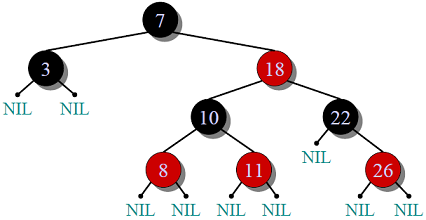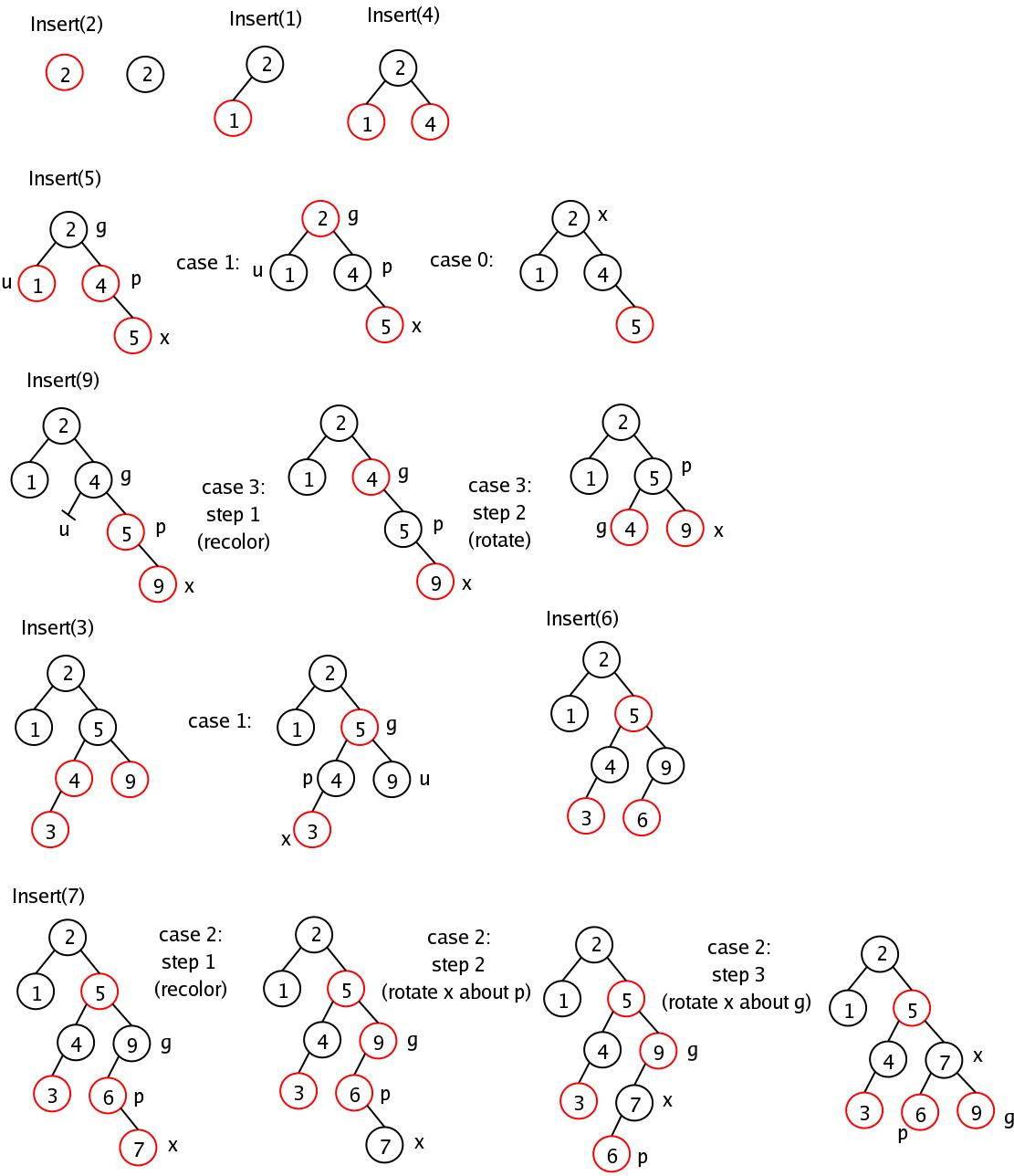×

Search anything:

# Red Black Tree: Insertion

#### red black tree Data Structures tree data structure insertion operationGet this book -> Problems on Array: For Interviews and Competitive Programming

Reading time: 15 minutes | Coding time: 9 minutes

A red–black tree is a kind of self-balancing binary search tree in computer science. Each node of the binary tree has an extra bit, and that bit is often interpreted as the color (red or black) of the node. These color bits are used to ensure the tree remains approximately balanced during insertions and deletions.

We will explore the insertion operation on a Red Black tree in the session. Inserting a value in Red Black tree takes O(log N) time complexity and O(N) space complexity.

• In addition to the requirements imposed on a binary search tree the following must be satisfied by a red–black tree:
• Each node is either red or black.
• The root is black. This rule is sometimes omitted. Since the root can always be changed from red to black, but not necessarily vice versa, this rule has little effect on analysis.
• All leaves (NIL) are black.
• If a node is red, then both its children are black.
• Every path from a given node to any of its descendant NIL nodes contains the same number of black nodes.The figure depicts the basic structure of Red Black Tree.

### Algorithm

• Basic operations associated with Red Black Tree:
• To add an element to a Red Black Tree, we must follow this algorithm:

• 1) Check whether tree is Empty.
• 2) If tree is Empty then insert the newNode as Root node with color Black and exit from the operation.
• 3) If tree is not Empty then insert the newNode as a leaf node with Red color.
• 4) If the parent of newNode is Black then exit from the operation.
• 5) If the parent of newNode is Red then check the color of parent node's sibling of newNode.
• 6) If it is Black or NULL node then make a suitable Rotation and Recolor it.
• 7) If it is Red colored node then perform Recolor and Recheck it. Repeat the same until tree becomes Red Black Tree.

•The figure illustrates the insertion of an element in a Red Black Tree.

### Complexity

• Average and Worst case insertion time complexity: `Θ(log n)`

• Average and Worst case Space complexity: `Θ(n)`

### Pseudocode

Insertion in a Red Black Tree
``````
y ← null
x ← T->root
while x ≠ null
do y ← x
if z->key < x->key
then x ← x->left
else x ← x->right
z->p ← y
if y = null
then T->root ← z
else if z->key < y->key
then y->left ← z
else y->right ← z
z->left ← null
z->right ← null
z->color ← RED
``````RB-INSERT-FIXUP(T, z)
while z->p->color = RED
do if z->p = z->p->p->left
then y ← z->p->p->right
if y->color = RED
then z->p->color ← BLACK Case 1
y->color ← BLACK Case 1
z->p->p->color ← RED Case 1
z ← z->p->p Case 1
else if z = z->p->right
then z ← z->p Case 2
LEFT-ROTATE(T, z) Case 2
z->p->color ← BLACK Case 3
z->p->p->color ← RED Case 3
RIGHT-ROTATE(T, z->p->p) Case 3
else (same as then clause with "right" and "left" exchanged)
T->root->color ← BLACK
``````

### Implementations

Implementations of Red Black tree is as follows:

• C++

### C++

``````
#include <iostream>
#include <cstdio>
#include <cstring>
#include <algorithm>
#include <cmath>
#include <vector>
#include <cstdlib>
#include <cassert>
#define INDENT_STEP  4
using namespace std;
enum color { RED, BLACK };
/*
* Node RBTree Declaration
*/
typedef struct rbtree_node
{
enum color color;
void *key;
void *value;
rbtree_node *left, *right, *parent;
}*node;
typedef struct rbtree_t
{
node root;
}*rbtree;
/*
* Class RBTree Declaration
*/
class RBTree
{
public:
typedef int (*compare_func)(void* left, void* right);
rbtree rbtree_create();
void* rbtree_lookup(rbtree t, void* , compare_func compare);
void rbtree_insert(rbtree t, void* , void* , compare_func compare);
void rbtree_delete(rbtree t, void* , compare_func compare);
node grandparent(node n);
node sibling(node n);
node uncle(node n);
void verify_properties(rbtree t);
void verify_property_1(node root);
void verify_property_2(node root);
color node_color(node n);
void verify_property_4(node root);
void verify_property_5(node root);
void verify_property_5_helper(node n, int , int*);
node new_node(void* key, void* , color , node , node);
node lookup_node(rbtree t, void* , compare_func compare);
void rotate_left(rbtree t, node n);
void rotate_right(rbtree t, node n);
void replace_node(rbtree t, node oldn, node newn);
void insert_case1(rbtree t, node n);
void insert_case2(rbtree t, node n);
void insert_case3(rbtree t, node n);
void insert_case4(rbtree t, node n);
void insert_case5(rbtree t, node n);
node maximum_node(node root);
void delete_case1(rbtree t, node n);
void delete_case2(rbtree t, node n);
void delete_case3(rbtree t, node n);
void delete_case4(rbtree t, node n);
void delete_case5(rbtree t, node n);
void delete_case6(rbtree t, node n);
};
/*
* Return Grandparent of Node
*/
node RBTree::grandparent(node n)
{
assert (n != NULL);
assert (n->parent != NULL);
assert (n->parent->parent != NULL);
return n->parent->parent;
}
/*
* Return Sibling of Node
*/
node RBTree::sibling(node n)
{
assert (n != NULL);
assert (n->parent != NULL);
if (n == n->parent->left)
return n->parent->right;
else
return n->parent->left;
}
/*
* Return Uncle of Node
*/
node RBTree::uncle(node n)
{
assert (n != NULL);
assert (n->parent != NULL);
assert (n->parent->parent != NULL);
return sibling(n->parent);
}
/*
* Verifying Properties of Red black Tree
*/
void RBTree::verify_properties(rbtree t)
{
verify_property_1 (t->root);
verify_property_2 (t->root);
verify_property_4 (t->root);
verify_property_5 (t->root);
}
/*
* Verifying Property 1
*/
void RBTree::verify_property_1(node n)
{
assert (node_color(n) == RED || node_color(n) == BLACK);
if (n == NULL)
return;
verify_property_1(n->left);
verify_property_1(n->right);
}
/*
* Verifying Property 2
*/
void RBTree::verify_property_2(node root)
{
assert (node_color(root) == BLACK);
}
/*
* Returns color of a node
*/
color RBTree::node_color(node n)
{
return n == NULL ? BLACK : n->color;
}
/*
* Verifying Property 4
*/
void RBTree::verify_property_4(node n)
{
if (node_color(n) == RED)
{
assert (node_color(n->left) == BLACK);
assert (node_color(n->right) == BLACK);
assert (node_color(n->parent) == BLACK);
}
if (n == NULL)
return;
verify_property_4(n->left);
verify_property_4(n->right);
}
/*
* Verifying Property 5
*/
void RBTree::verify_property_5(node root)
{
int black_count_path = -1;
verify_property_5_helper(root, 0, &black_count_path);
}
void RBTree::verify_property_5_helper(node n, int black_count, int* path_black_count)
{
if (node_color(n) == BLACK)
{
black_count++;
}
if (n == NULL)
{
if (*path_black_count == -1)
{
*path_black_count = black_count;
}
else
{
assert (black_count == *path_black_count);
}
return;
}
verify_property_5_helper(n->left,  black_count, path_black_count);
verify_property_5_helper(n->right, black_count, path_black_count);
}
/*
* Create Red Black Tree
*/
rbtree RBTree::rbtree_create()
{
rbtree t = new rbtree_t;
t->root = NULL;
verify_properties(t);
return t;
}
/*
* Creating New Node of Reb Black Tree
*/
node RBTree::new_node(void* k, void* v, color n_color, node left, node right)
{
node result = new rbtree_node;
result->key = k;
result->value = v;
result->color = n_color;
result->left = left;
result->right = right;
if (left  != NULL)
left->parent = result;
if (right != NULL)
right->parent = result;
result->parent = NULL;
return result;
}
/*
* Look Up through Node
*/
node RBTree::lookup_node(rbtree t, void* key, compare_func compare)
{
node n = t->root;
while (n != NULL)
{
int comp_result = compare(key, n->key);
if (comp_result == 0)
{
return n;
}
else if (comp_result < 0)
{
n = n->left;
}
else
{
assert(comp_result > 0);
n = n->right;
}
}
return n;
}
/*
* RbTree Look Up
*/
void* RBTree::rbtree_lookup(rbtree t, void* key, compare_func compare)
{
node n = lookup_node(t, key, compare);
return n == NULL ? NULL : n->value;
}
/*
* Rotate left
*/
void RBTree::rotate_left(rbtree t, node n)
{
node r = n->right;
replace_node(t, n, r);
n->right = r->left;
if (r->left != NULL)
{
r->left->parent = n;
}
r->left = n;
n->parent = r;
}
/*
* Rotate right
*/
void RBTree::rotate_right(rbtree t, node n)
{
node L = n->left;
replace_node(t, n, L);
n->left = L->right;
if (L->right != NULL)
{
L->right->parent = n;
}
L->right = n;
n->parent = L;
}
/*
* Replace a node
*/
void RBTree::replace_node(rbtree t, node oldn, node newn)
{
if (oldn->parent == NULL)
{
t->root = newn;
}
else
{
if (oldn == oldn->parent->left)
oldn->parent->left = newn;
else
oldn->parent->right = newn;
}
if (newn != NULL)
{
newn->parent = oldn->parent;
}
}
/*
* Insert node into RBTree
*/
void RBTree::rbtree_insert(rbtree t, void* key, void* value, compare_func compare)
{
node inserted_node = new_node(key, value, RED, NULL, NULL);
if (t->root == NULL)
{
t->root = inserted_node;
}
else
{
node n = t->root;
while (1)
{
int comp_result = compare(key, n->key);
if (comp_result == 0)
{
n->value = value;
return;
}
else if (comp_result < 0)
{
if (n->left == NULL)
{
n->left = inserted_node;
break;
}
else
{
n = n->left;
}
}
else
{
assert (comp_result > 0);
if (n->right == NULL)
{
n->right = inserted_node;
break;
}
else
{
n = n->right;
}
}
}
inserted_node->parent = n;
}
insert_case1(t, inserted_node);
verify_properties(t);
}
/*
* Inserting Case 1
*/
void RBTree::insert_case1(rbtree t, node n)
{
if (n->parent == NULL)
n->color = BLACK;
else
insert_case2(t, n);
}
/*
* Inserting Case 2
*/
void RBTree::insert_case2(rbtree t, node n)
{
if (node_color(n->parent) == BLACK)
return;
else
insert_case3(t, n);
}
/*
* Inserting Case 3
*/
void RBTree::insert_case3(rbtree t, node n)
{
if (node_color(uncle(n)) == RED)
{
n->parent->color = BLACK;
uncle(n)->color = BLACK;
grandparent(n)->color = RED;
insert_case1(t, grandparent(n));
}
else
{
insert_case4(t, n);
}
}
/*
* Inserting Case 4
*/
void RBTree::insert_case4(rbtree t, node n)
{
if (n == n->parent->right && n->parent == grandparent(n)->left)
{
rotate_left(t, n->parent);
n = n->left;
}
else if (n == n->parent->left && n->parent == grandparent(n)->right)
{
rotate_right(t, n->parent);
n = n->right;
}
insert_case5(t, n);
}
/*
* Inserting Case 5
*/
void RBTree::insert_case5(rbtree t, node n)
{
n->parent->color = BLACK;
grandparent(n)->color = RED;
if (n == n->parent->left && n->parent == grandparent(n)->left)
{
rotate_right(t, grandparent(n));
}
else
{
assert (n == n->parent->right && n->parent == grandparent(n)->right);
rotate_left(t, grandparent(n));
}
}
/*
* Returns Maximum node
*/
node RBTree::maximum_node(node n)
{
assert (n != NULL);
while (n->right != NULL)
{
n = n->right;
}
return n;
}
/*
* Compare two nodes
*/
int compare_int(void* leftp, void* rightp)
{
int left = (int)leftp;
int right = (int)rightp;
if (left < right)
return -1;
else if (left > right)
return 1;
else
{
assert (left == right);
return 0;
}
}
/*
* Print RBTRee
*/
void print_tree_helper(node n, int indent)
{
int i;
if (n == NULL)
{
fputs("<empty tree>", stdout);
return;
}
if (n->right != NULL)
{
print_tree_helper(n->right, indent + INDENT_STEP);
}
for(i = 0; i < indent; i++)
fputs(" ", stdout);
if (n->color == BLACK)
cout<<(int)n->key<<endl;
else
cout<<"<"<<(int)n->key<<">"<<endl;
if (n->left != NULL)
{
print_tree_helper(n->left, indent + INDENT_STEP);
}
}
void print_tree(rbtree t)
{
print_tree_helper(t->root, 0);
puts("");
}
/*
*/
int main()
{
int i;
RBTree rbt;
rbtree t = rbt.rbtree_create();
for (i = 0; i < 12; i++)
{
int x = rand() % 10;
int y = rand() % 10;
print_tree(t);
cout<<"Inserting "<<x<<" -> "<<y<<endl<<endl;
rbt.rbtree_insert(t, (void*)x, (void*)y, compare_int);
assert(rbt.rbtree_lookup(t, (void*)x, compare_int) == (void*)y);
}
return 0;
}
``````

### Applications

Importance of Red Black Tree:
• Completely Fair Scheduler in Linux Kernel
• Computational Geometry Data structures
• In various implementations of Associative Data structures (for e.g C++ STL uses RB tree internally to implement Set and Map)